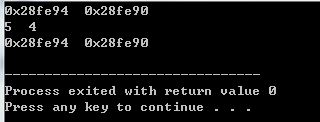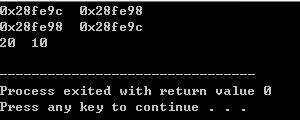# 1.指针形参：实现指针指向的值的交换（指针本身不变）

#include<iostream>
#include<string>
using namespace std;

void Swap(int *x,int *y)
{
int temp;
temp = *x;
*x = *y;
*y = temp;
}
int main()
{
int a = 4;
int b = 5;
int *x = &a;
int *y = &b;
cout<<x<<"  "<<y<<endl;
Swap(x,y);
cout<<*x<<"  "<<*y<<endl;
cout<<x<<"  "<<y<<endl;
return 0;
}# 2.传递指向指针的引用：实现两个指针的交换

#include<iostream>
using namespace std;

void ptrSwap(int *&x,int *&y)
{
int *tmp=y;
y=x;
x=tmp;
}
int main()
{
int a=10;
int b=20;
int *x=&a;
int *y=&b;
cout<<x<<"  "<<y<<endl;
ptrSwap(x,y);
cout<<x<<"  "<<y<<endl;
cout<<*x<<"  "<<*y<<endl;
return 0;
}